# Massless field

(diff) ← Older revision | Latest revision (diff) | Newer revision → (diff)

A quantum field theory is said to contain massless fields if in the Hilbert space generated by repeated application of the quantum fields to the vacuum state there exist subspaces associated with one-particle states of mass zero.

According to the concept of a relativistic particle, introduced by E. Wigner [a1], the one-particle states associated with a particle of type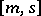are given by Hilbert subspaces transforming irreducibly under the unitary representation of the covering group of the orthochronous proper Poincaré group (cf. also Poincaré group)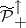. Here,is the eigenvalue of the states in these subspaces with respect to the mass operator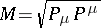, where the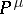(withreferring to the "time variable" ) are the generators of space-time translations (the four-vectoris also called the energy-momentum operator), and one finds thatis well-defined by the spectral condition that the (joint) spectrum oflies in the forward lightcone [a2]. Furthermore,stands for the spin associated with the representation of the little group, i.e. the subgroup instabilizing a vectorin the Minkowski space-time with Minkowski inner productand. This representation of the little group is furthermore assumed to be finite dimensional for subspaces associated with one-particle states.

In the massless case, the little group stabilizing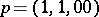is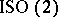and the finite-dimensional representations of this group are characterized by a number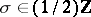called the helicity, which has the physical interpretation of the amount of the internal angular momentum (respectively, "spin" ) directed in the flight direction of the particle. The particle type associated with a massless particle is thus denoted by the pair.

Denote the field operators of the theory by, withfrom a suitable space of Schwartz test functions (cf. also Generalized functions, space of). If these field operators connect the vacuumof the theory with the one-particle states with label, i.e.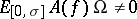(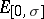being the projector associated with the one-particle Hilbert space of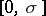-states), one can develop a scattering theory for the quantum field, following [a3], [a4]: For a test function, letwith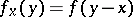. Take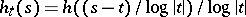, witha positive test function of compact support with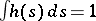and set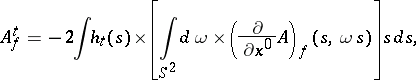whereis the unit sphere in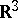andmeans integration over all unit vectorsin.

Then it was shown in the above-mentioned references (in the axiomatic framework described in [a2], [a3], [a4]), using the Reeh–Schlieder theorem [a2] together with a kind of Huygens principle and locality, that the ( "adiabatic" ) limit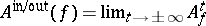can be defined on a suitable dense domain. The quantum fieldsare by definition the free asymptotic quantum fields associated to the field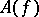. Repeated application of the field operators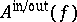to the vacuumgenerates the incoming and outgoing multiple particle states of particle type, which define in- and out-Fock spaces (cf. also Fock space). The scalar product of states from the in-Fock space with states from the out-Fock space define the scattering matrix for scattering processes, which only involve massless incoming and outgoing particles.

A number of mathematical problems and physical effects arise in the presence of massless fields, as, for example (see the literature for further details): massless fields are intimately connected with long-range forces in elementary particle physics such as e.g. electro-magnetism, see e.g. [a5]. Among others, this leads to mathematical problems in the theory of superselection sectors associated to the algebra of observables of a quantum field theory involving long-range forces [a6].

In the quantum field theory of gauge fields, massless gauge fields are being coupled to Fermionic currents by the Gauss law, which makes it necessary to introduce an indefinite inner product on the state space underlying the quantum field theory and to single out a subspace of "physical states" with positive norm by a gauge principle [a7], [a8], [a9] (for models partly implementing this, see [a10], [a11]).

If massive particles (cf. also Massive field) interact with massless particles, the massive particle can be accompanied by a "cloud" of infinitely many massless particles with finite total energy, which can "smear out" the mass of the massive particle and give rise to the infraparticle problem [a12], [a13]. In the perturbation theory of quantum fields this effect is assumed to justify the mass renormalization, cf. [a5]. Furthermore, mass-zero particles in perturbation theory cause infrared divergences and the problem of summing up Feynman graphs of all orders with "soft" (i.e. low-energy) massless particles [a5].

The occurrence of so-called Goldstone bosons, which are massless particles, is related to symmetry breaking in quantum field theory; for two different aspects of this phenomenon, see e.g. [a5], Vol. II, [a14].

How to Cite This Entry:
Massless field. Encyclopedia of Mathematics. URL: http://encyclopediaofmath.org/index.php?title=Massless_field&oldid=16672
This article was adapted from an original article by S. AlbeverioH. Gottschalk (originator), which appeared in Encyclopedia of Mathematics - ISBN 1402006098. See original article